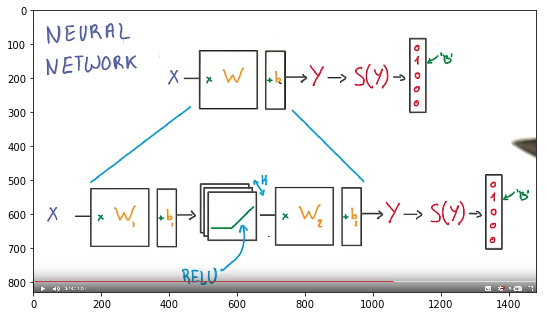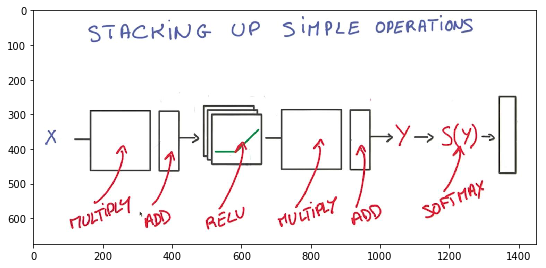## one hidden layer neural network¶

• with
• regularization
• dropout
In :
!nvcc --version
!python -V

nvcc: NVIDIA (R) Cuda compiler driver
Built on Mon_Jan__9_17:32:33_CST_2017
Cuda compilation tools, release 8.0, V8.0.60

Python 3.5.2 :: Anaconda 4.3.0 (64-bit)


official tutorial

https://www.tensorflow.org/tutorials/

Learning notebook of Udacity's deep learning course

https://www.udacity.com/course/deep-learning--ud730

Install Tensorflow https://www.tensorflow.org/install/

• adviced to have a NVIDIA GPU, mine is GTX1060
• I've successfully installed Tensorflow on Windows 7
• install CUDA® Toolkit 8.0

### notMNIST dataset¶

http://yaroslavvb.blogspot.com/2011/09/notmnist-dataset.html

In :
import tensorflow as tf
import numpy as np
import pandas as pd
import matplotlib.pyplot as plt

from six.moves import cPickle as pickle
from sklearn.metrics import accuracy_score, classification_report
%matplotlib inline
tf.__version__

Out:
'1.0.0'
In :
pickle_file = 'notMNIST.pickle'

with open(pickle_file, 'rb') as f:
train_dataset = save['train_dataset']
train_labels = save['train_labels']
valid_dataset = save['valid_dataset']
valid_labels = save['valid_labels']
test_dataset = save['test_dataset']
test_labels = save['test_labels']
del save  # hint to help gc free up memory
print('Training set', train_dataset.shape, train_labels.shape)
print('Validation set', valid_dataset.shape, valid_labels.shape)
print('Test set', test_dataset.shape, test_labels.shape)

Training set (480000, 28, 28) (480000,)
Validation set (40000, 28, 28) (40000,)
Test set (18500, 28, 28) (18500,)


### explore data¶

In :
plt.figure(figsize=(10,10))
print (train_labels[:12])
for i in range(12):
plt.subplot(1,12,i+1)
plt.imshow(train_dataset[i], cmap='gray')
plt.axis('off')

[2 2 9 2 8 0 6 8 9 7 0 1]### tensorflow only accepts onehot label¶

Reformat into a shape that's more adapted to the models we're going to train:

• data as a flat matrix,
• labels as float 1-hot encodings.
In :
valid_labels

Out:
array([1, 5, 0, ..., 3, 9, 1])
In :
image_size = 28
num_labels = 10

def reformat(dataset, labels):
dataset = dataset.reshape((-1, image_size * image_size)).astype(np.float32)

# Map 0 to [1.0, 0.0, 0.0 ...], 1 to [0.0, 1.0, 0.0 ...]
labels = pd.get_dummies(labels).values.astype(np.float32)
#   labels = (np.arange(num_labels) == labels[:,None]).astype(np.float32)
return dataset, labels
train_dataset, train_labels = reformat(train_dataset, train_labels)
valid_dataset, valid_labels = reformat(valid_dataset, valid_labels)
test_dataset, test_labels = reformat(test_dataset, test_labels)
print('Training set', train_dataset.shape, train_labels.shape)
print('Validation set', valid_dataset.shape, valid_labels.shape)
print('Test set', test_dataset.shape, test_labels.shape)

Training set (480000, 784) (480000, 10)
Validation set (40000, 784) (40000, 10)
Test set (18500, 784) (18500, 10)


### build model structure¶

In :
plt.figure(figsize=(9,9))

Out:
<matplotlib.image.AxesImage at 0xe62c358>In :
plt.figure(figsize=(9,9))

Out:
<matplotlib.image.AxesImage at 0xea016d8>### make sure to train the train_dataset once¶

In :
print (train_dataset.shape)
batch_size = 32
num_steps = 40001

for i in range(num_steps):
c = ( i * batch_size) % (train_labels.shape - batch_size)
if c == 0:
print ('circle', end=' ')

480000
circle circle circle 

## Create notes over a computation graph¶

### evaluation¶

• accuracy
In :
def accuracy(labels, predictions):
from sklearn.metrics import accuracy_score

percent = accuracy_score(
np.argmax(labels,1), np.argmax(predictions,1)
)*100

return percent


### add L2 regularization and dropout¶

• dropout in kernel func
• L2 in loss func
In :
batch_size = 128
hidden_nodes = 8192
beta = 0.001
learning_rate = 0.1


### learning rate decay¶

In :
### specify a graph
graph = tf.Graph()

with graph.as_default():

step = tf.Variable(0, trainable=False)  # count the number of steps taken.

#     learning_rate = tf.train.exponential_decay(0.25, global_step, 4000, 0.96, staircase=True)

keep = tf.placeholder(tf.float32)
# Input data.
# For the training data, we use a placeholder that will be fed.
tf_train_dataset = tf.placeholder(tf.float32, shape = (None, image_size * image_size))
tf_train_labels = tf.placeholder(tf.float32, shape = (None, num_labels))

# valid dataset
# Load the validation data into constants that are attached to the graph.
tf_valid_dataset = tf.constant(valid_dataset)
# Load the test data into constants that are attached to the graph.
tf_test_dataset = tf.constant(test_dataset)

# Variables.
# These are the parameters that we are going to be training.
# The weight matrix will be initialized using random values following a (truncated) normal distribution.
# The biases get initialized to zero.

# the shape of weights are very important
# weight1 for every row (each train instance), column should be 28*28

# so matrix(input) shape batch*784 [matrix factorization] 784*hidden_nodes (weight1) = (matrix) batch*hidden_notes ---> relu
# then matrix relu--> shape batch*hidden_notes [matrix multiply] weight2: hidden_notes*labels(10) = batch * labels(10)

# and biases1 shape is hidden_notes * 1
# biases2 's shape is labels(10) * 1

weights1 = tf.Variable(
tf.truncated_normal([image_size * image_size, hidden_nodes]))

biases1 = tf.Variable(tf.zeros([hidden_nodes]))

weights2 = tf.Variable(
tf.truncated_normal([hidden_nodes, num_labels]))

biases2 = tf.Variable(tf.zeros([num_labels]))

# Training computation.
# We multiply the inputs with the weight matrix, and add biases.
# We compute the softmax and cross-entropy
# (it's one operation in TensorFlow, because it's very common, and it can be optimized).
# We take the average of this cross-entropy across all training examples: that's our loss.

# tf.matul(t1, t2): matrix multiply

def forward_prop(in_put):

h1 = tf.nn.relu(tf.matmul(in_put, weights1) + biases1)

h1 = tf.nn.dropout(h1, keep_prob= keep)

out_put = tf.matmul(h1, weights2) + biases2

return out_put

logits = forward_prop(tf_train_dataset)

# tf.reduce_mean(t): Computes the mean of elements across dimensions of a tensor.
# tf.nn.softmax_cross_entropy_with_logits(labels = , logits = ): Computes softmax cross entropy between logits and labels.

loss = tf.reduce_mean(
tf.nn.softmax_cross_entropy_with_logits( labels = tf_train_labels, logits = logits )
+ beta * (tf.nn.l2_loss(weights1) + tf.nn.l2_loss(weights2)
+ tf.nn.l2_loss(biases1) + tf.nn.l2_loss(biases2))
)

# Optimizer.
# We are going to find the minimum of this loss using gradient descent.
optimizer = tf.train.GradientDescentOptimizer(learning_rate = learning_rate).minimize(loss = loss, global_step = step)

# Predictions for the training, validation, and test data.
# These are not part of training, but merely here so that we can report accuracy figures as we train.
train_prediction = tf.nn.softmax(logits)

valid_prediction = tf.nn.softmax(forward_prop(tf_valid_dataset))
test_prediction = tf.nn.softmax(forward_prop(tf_test_dataset))


• create a Placeholder node which will be fed actual data at every call of session.run().

tf.global_variables_initializer().run()

• Run the named file inside IPython as a program.
In :
num_steps = 80001

In :
from time import time
start = time()

with tf.Session(graph=graph) as session:
tf.global_variables_initializer().run()
print("Initialized")

for step in range(num_steps):

# Pick an offset within the training data, which has been randomized.
# Note: we could use better randomization across epochs.
offset = (step * batch_size) % (train_labels.shape - batch_size)

# Generate a minibatch.
batch_data = train_dataset[offset:(offset + batch_size), :]
batch_labels = train_labels[offset:(offset + batch_size), :]

# Prepare a dictionary telling the session where to feed the minibatch.
# The key of the dictionary is the placeholder node of the graph to be fed,
# and the value is the numpy array to feed to it.
feed_dict = {tf_train_dataset : batch_data,
tf_train_labels : batch_labels,
keep: 0.5}

_, l, predictions = session.run([optimizer, loss, train_prediction], feed_dict=feed_dict)

if step ==0:
stepping = 1000

if (step % stepping == 0):

va_pred, va_real = valid_prediction.eval(feed_dict={keep: 1.0}), valid_labels
va_acc = accuracy(va_pred, va_real)

pred, real = test_prediction.eval(feed_dict={keep: 1.0}), test_labels
te_acc = accuracy(pred, real)

if (step % 8000 == 0):

print('\ntime passed: %.2f s'%(time()-start))
print("Minibatch loss at step %d: %f" % (step, l))
print("Minibatch accuracy: %.1f%%" % accuracy(batch_labels, predictions))

print("Validation accuracy: %.1f%%" % va_acc)
print("Test accuracy: %.1f%%" % te_acc)

if va_acc > 89.5 and (step % 800 == 0):
print("Minibatch loss at step %d: %f: high score" % (step, l))

print("Validation accuracy: %.1f%%" % va_acc)
print("Test accuracy: %.1f%%" % te_acc)

if va_acc > 87 and stepping > 100:
stepping = 100

if va_acc > 89.5:
stepping = 5

if va_acc >= 90:
print ('\nfind a good one!')
print("Minibatch loss at step %d: %f: highest score" % (step, l))
print("Validation accuracy: %.1f%%" % va_acc)
break

pred, real = test_prediction.eval(feed_dict={keep: 1.0}), test_labels
print("Final Test accuracy: %.1f%%" % accuracy(pred, real))

print ('\ndone')
print('\ntotal time: %.2f s'%(time()-start))

Initialized

time passed: 1.51 s
Minibatch loss at step 0: 3965.524902
Minibatch accuracy: 7.8%
Validation accuracy: 27.9%
Test accuracy: 30.6%

time passed: 96.52 s
Minibatch loss at step 8000: 506.893372
Minibatch accuracy: 78.9%
Validation accuracy: 88.0%
Test accuracy: 93.9%

time passed: 199.95 s
Minibatch loss at step 16000: 100.640472
Minibatch accuracy: 92.2%
Validation accuracy: 88.4%
Test accuracy: 94.2%
Minibatch loss at step 20000: 45.267906: high score
Validation accuracy: 89.5%
Test accuracy: 95.1%
Minibatch loss at step 21600: 32.875980: high score
Validation accuracy: 89.8%
Test accuracy: 95.4%
Minibatch loss at step 22400: 28.120575: high score
Validation accuracy: 89.9%
Test accuracy: 95.5%

find a good one!
Minibatch loss at step 22855: 25.631189: highest score
Validation accuracy: 90.1%
Final Test accuracy: 95.5%

done

total time: 679.52 s


Validation accuracy: 88.2%

Test accuracy: 94.0%

In :
letters = list('ABCDEFGHIJK')
dic = {i: letters[i] for i in range(len(letters))}
print(dic)

{0: 'A', 1: 'B', 2: 'C', 3: 'D', 4: 'E', 5: 'F', 6: 'G', 7: 'H', 8: 'I', 9: 'J', 10: 'K'}

In :
plt.figure(figsize=(10,10))
for i in range(12):
plt.subplot(1,12,i+1)
plt.imshow(test_dataset[i].reshape(28,28), cmap='gray')
plt.axis('off')## predict using the trained model¶

In :
for i in pd.Series(pred.argmax(1)).map(dic)[:12]:print (i, end=' ')

C D J J F G J E A A E D

### real label¶

In :
for i in pd.Series(real.argmax(1)).map(dic)[:12]:print (i, end=' ')

C D J J F G J E A A E D
In [ ]: